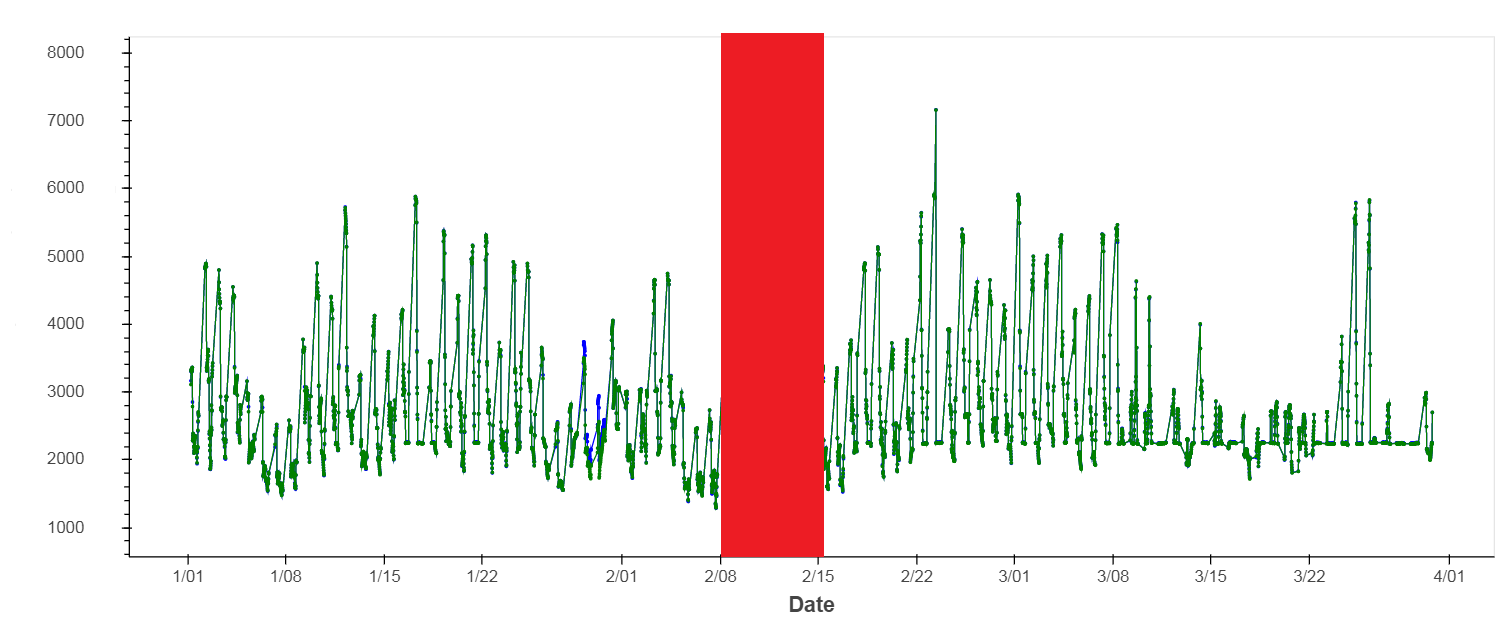# bokeh patch with datetime axis

Dear Bokeh group,

I currently have a figure created with the following code:

p = figure(plot_width = 1200, plot_height = 500,

x_axis_type = “datetime”, x_axis_label = “Date”,

y_axis_label = self._var_dict.get(self.y_name),

toolbar_location = “above”, tools = tool_l,

y_range = Range1d(yrange_min, yrange_max))

#create line plots

l1 = p.line(“date”, “y”, source = source, color = “blue”,

legend = self._var_dict.get(self.y_name) + " (data)")

c1 = p.circle(“date”, “y”, source = source, color = “blue”,

size = 2,

legend = self._var_dict.get(self.y_name) + " (data)")

However I would like to add a patch on top of it, I understand the example on documentation: p.patch([1, 2, 3, 4, 5], [6, 7, 8, 7, 3], alpha=0.5, line_width=2), but how does it work with an x aixs with type “datetime”?Thanks!

Dear group, can anyone please look at this question and help me out?

Thank you so much!

···

On Tuesday, October 17, 2017 at 4:42:16 PM UTC-5, Qinpeng Wang wrote:

Dear Bokeh group,

I currently have a figure created with the following code:

p = figure(plot_width = 1200, plot_height = 500,

x_axis_type = “datetime”, x_axis_label = “Date”,

y_axis_label = self._var_dict.get(self.y_name),

toolbar_location = “above”, tools = tool_l,

y_range = Range1d(yrange_min, yrange_max))

#create line plots

l1 = p.line(“date”, “y”, source = source, color = “blue”,

legend = self._var_dict.get(self.y_name) + " (data)")

c1 = p.circle(“date”, “y”, source = source, color = “blue”,

size = 2,

legend = self._var_dict.get(self.y_name) + " (data)")

However I would like to add a patch on top of it, I understand the example on documentation: p.patch([1, 2, 3, 4, 5], [6, 7, 8, 7, 3], alpha=0.5, line_width=2), but how does it work with an x aixs with type “datetime”?Thanks!

Hi,

I’m not sure what your question is. It just does. Just specify its “x” as the datetime data - either as explicit values or as a data source, just as you used a source for your line and circle glyphs.

Also, if all you need is a vertical bar spanning your whole plot, I’d suggest using BoxAnnotation: https://bokeh.pydata.org/en/latest/docs/user_guide/annotations.html#box-annotations

Regards,

Eugene

···

On Thursday, October 26, 2017 at 11:03:36 PM UTC+7, Qinpeng Wang wrote:

Dear group, can anyone please look at this question and help me out?

Thank you so much!

On Tuesday, October 17, 2017 at 4:42:16 PM UTC-5, Qinpeng Wang wrote:

Dear Bokeh group,

I currently have a figure created with the following code:

p = figure(plot_width = 1200, plot_height = 500,

x_axis_type = “datetime”, x_axis_label = “Date”,

y_axis_label = self._var_dict.get(self.y_name),

toolbar_location = “above”, tools = tool_l,

y_range = Range1d(yrange_min, yrange_max))

#create line plots

l1 = p.line(“date”, “y”, source = source, color = “blue”,

legend = self._var_dict.get(self.y_name) + " (data)")

c1 = p.circle(“date”, “y”, source = source, color = “blue”,

size = 2,

legend = self._var_dict.get(self.y_name) + " (data)")

However I would like to add a patch on top of it, I understand the example on documentation: p.patch([1, 2, 3, 4, 5], [6, 7, 8, 7, 3], alpha=0.5, line_width=2), but how does it work with an x aixs with type “datetime”?Thanks!